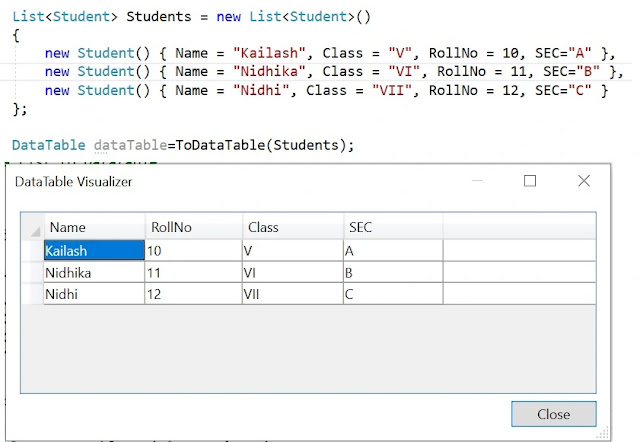### Convert a List to C# Datatable in Csharp

Kailash Chandra Behera | Saturday, September 17, 2022### Introduction

There is now specific function provided by Microsoft Csharp which will convert a list to C# Datatable. Here this blog provides a code example that convert a list to Datatable.

### Getting Started

There are various ways to convert a list to Datatable, you can find in various blog sites. Here in this blog we will see how to convert using C# Reflection. While converting a type of collection to other type of collection, casting data is an complex task.

Reflection is a very simple and convenient way where you need not think about casting of data, because at runtime, we can know which properties values need to be converted which data type.

For example, while converting a list the data type of each Colum value or which data type the Datatable accepts can be known at run time. Let’s see the code blocks below to get more about it but before that I want give you a little details about C# reflection for better understanding of code.

Reflection Meaning:

A Reflection provides objects (of type Type) that describe assemblies, modules, and types. You can use reflection to dynamically create an instance of a type, bind the type to an existing object, or get the type from an existing object and invoke its methods or access its fields and properties. If you are using attributes in your code, reflection enables you to access them. For more information, see Attributes.

Reflection is useful in the following situations:

1. When you have to access attributes in your program's metadata. For more information, see Retrieving Information Stored in Attributes.
2. For examining and instantiating types in an assembly.
3. For building new types at run time. Use classes in System.Reflection.Emit.
4. For performing late binding, accessing methods on types created at run time. See the topic Dynamically Loading and Using Types.
Demonstration

Here’s below are simple examples which descriobes to convert a list to Datatable using reflection. The below code blocks declare a class named student to store student information.

`````` public class Student
{
//StudentName
public string Name { get; set; }
public int RollNo { get; set; }
public string Class { get; set; }
//Section
public string SEC { get; set; }
}
``````

Following block declares a list and stored student information into the collection(List).

`````` static void Main(string[] args)
{
List<Student> Students = new List<Student>()
{
new Student() { Name = "Kailash", Class = "V", RollNo = 10, SEC="A" },
new Student() { Name = "Nidhika", Class = "VI", RollNo = 11, SEC="B" },
new Student() { Name = "Nidhi", Class = "VII", RollNo = 12, SEC="C" }
};
DataTable dataTable=ToDataTable(Students);
}
``````

The function ToDataTable in below code blocks, uses c# refelection to convert a list to Datatable. See this codes carefully it contail actuall logic to convert. First the code block retrieves list of properties and creates datatable columns based on on the property name and datatype. Then it retrieves list of values from collection and insterts into the table.

`````` public static DataTable ToDataTable<T>(List<T> items)
{
//c# datatable
DataTable dataTable = new DataTable(typeof(T).Name);
//Get all the properties
PropertyInfo[] Props = typeof(T).GetProperties(BindingFlags.Public | BindingFlags.Instance);
foreach (PropertyInfo prop in Props)
{
//Setting column name and datatype of DataTable same as to Property names
DataColumn dataColumn =new DataColumn();
dataColumn.ColumnName = prop.Name;
dataColumn.DataType = prop.PropertyType;
}
//fetchine list of values to insert
foreach (T item in items)
{
//array of object to store property values
dynamic values = new dynamic[Props.Length];
DataRow dataRow=dataTable.NewRow();
for (int i = 0; i < Props.Length; i++)
{
//inserting list of values to datatable rows
dataRow[i] = Convert.ChangeType(Props[i].GetValue(item, null), Props[i].PropertyType);
}